# Ex.3.3 Q2 Understanding Quadrilaterals Solution-Ncert Maths Class 8

## Question

Consider the following parallelograms. Find the values of the unknowns $$x$$, $$y$$, $$z$$.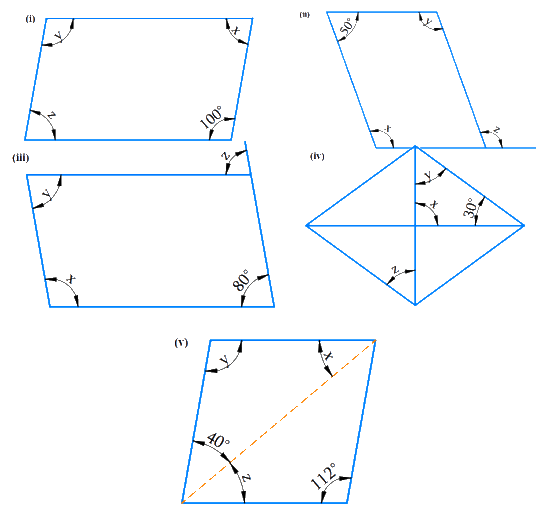Video Solution
Understanding Quadrilaterals
Ex 3.3 | Question 2

## Text Solution

i) What is Known?

$$ABCD$$ is a parallelogram.

What is Unknown?

Values of $$x$$, $$y$$, $$z$$.

Reasoning:

In a parallelogram, opposite angles are equal and adjacent angles are supplementary. Using this property, we can calculate the measure of unknown angles.

Steps: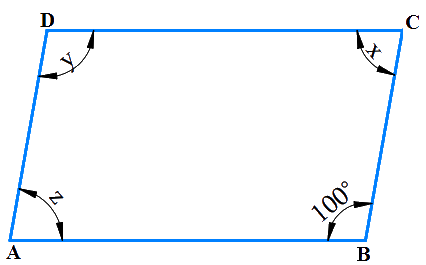Since $$D$$ is opposite to $$B$$.

So, $${{y }} = {\rm{ 1}}{00^{\rm{o}}}$$ [Since opposite angles of a parallelogram are equal]

$$\angle C + \angle {\rm{B}} = {\rm{18}}0^\circ$$(The adjacent angles in a parallelogram are supplementary)

$${{x }} + {\rm{ 1}}00^\circ {\rm{ }} = {\rm{ 18}}0^\circ {\rm{ }}$$ (The adjacent angles in a parallelogram are supplementary)

Therefore,

\begin{align} x & ={{180}^{{}^\circ }}-{{100}^{{}^\circ }} \\ {} & ={{80}^{{}^\circ }} \\\end{align}

$${{x}} = {{ z}} = {\rm{8}}0^\circ {\rm{ }}$$ [Since opposite angles of a parallelogram are equal]

ii) What is Known?

Given figure is a parallelogram.

What is Unknown?

values of $$x$$, $$y$$, $$z$$.

Reasoning:

In a parallelogram, opposite angles are equal and adjacent angles are supplementary. Using this property, we can calculate the measure of the unknown angles.

Steps: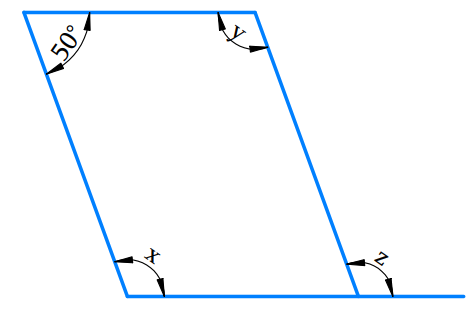\begin{align}{{\text{x}} + {\rm{5}}{0^\circ }}&{ = {\rm{18}}{0^\circ }\left( \begin{array}{l}{\text{The adjacent angles}}\\{\text{ in a parallelogram }}\\{\text{are supplementary}}\end{array} \right)}\\ {\rm{x}}&{ = {\rm{18}}{0^\circ } - {\rm{5}}{0^\circ }}\\&{ = {\rm{13}}{0^\circ }}\end{align}

\begin{align} & \text{x }\!=\!\text{y}\!=\!\text{13}0^\circ \left( \begin{array}{l}{\text{Since opposite angles}}\\{\text{ of a parallelogram }}\\{\text{are equal}}\end{array} \right) \\& \text{x}=\text{z}=\text{13}0^\circ \text{(Corresponding angles)} \\\end{align}

iii) What is Known?

Given figure is a parallelogram.

What is Unknown?

values of $$x$$, $$y$$, $$z$$.

Reasoning:

In a parallelogram, opposite angles are equal and adjacent angles are supplementary. Using this property, we can calculate the measure of the unknown angles

Steps: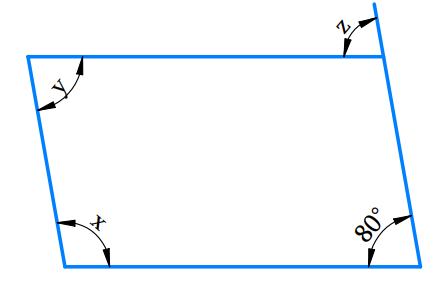\begin{align}z&={{80}^{\text{o}}}\text{ (Corresponding angles) } \\y&={{80}^{\text{o}}}\left( \begin{array}{l}{\text{since opposite angles}}\\{\text{ of a parallelogram }}\\{\text{are equal}}\end{array} \right) \\x+y&={{180}^{\text{o}}}\left( \begin{array}{l}{\text{Adjacent angles }}\\{\text{are supplementary}}\end{array} \right)\\x+{{80}^{\text{o}}}&={{180}^{\text{o}}} \\x&={{180}^{\text{o}}}-{{80}^{\text{o}}} \\x&={{100}^{{}^\circ }} \\\end{align}

$\text{Therefore x}={{100}^{{}^\circ }},\,\,\,\text{y}={{80}^{{}^\circ }},\,\,\,\text{z}={{80}^{{}^\circ }}$

iv) What is the known?

Given figure is a parallelogram.

What is unknown?

Values of $$x$$, $$y$$, $$z$$.

Reasoning:

In a parallelogram, opposite angles are equal and adjacent angles are supplementary. Using this property, we can calculate the measure of the unknown angles.

Steps: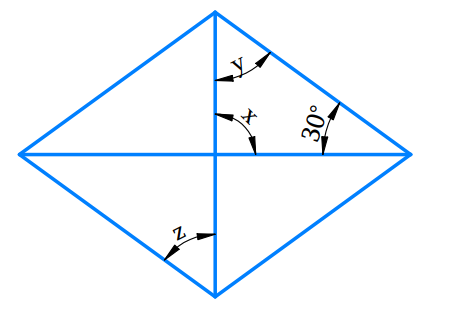\begin{align}\text{x}+\text{y}+{{30}^{\text{o}}}&={{180}^{\text{o}}}\left( \begin{array}{l}{\text{Angle sum}}\\{\text{ property of }}\\{\text{triangles}}\end{array} \right)\\ \text{x}&\!\!=\!\!{{90}^{\text{o}}}\left( \begin{array}{l}{\text{Vertically }}\\{\text{opposite angles}}\end{array} \right)\\ {{90}^{{}^\circ }}+\text{y}+{{30}^{\text{o}}}&={{180}^{\text{o}}} \\ \text{y}+120&={{180}^{\text{o}}} \\ \text{y}&={{180}^{\text{o}}} \\ \text{z}&={{60}^{\text{o}}}\end{align}

Therefore

$\text{z}=\text{y}={{60}^{\text{o}}}\left( \begin{array}{l}{\text{Alternate interior }}\\{\text{angles are equal}}\end{array} \right)$

v) What is Known?

Given figure is a parallelogram.

What is Unknown?

Values of $$x$$, $$y$$, $$z$$.

Reasoning:

In parallelogram opposite angles are equal and Adjacent angles are supplementary.

Using this property, we can calculate the unknown angles.

Steps: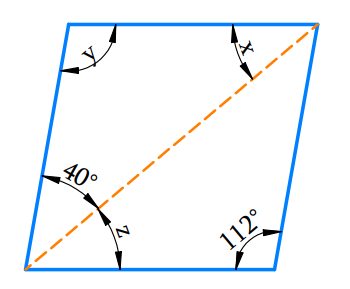$$y=112^\circ$$(Since opposite angles of a parallelogram are equal)

\begin{align}x+y+40^\circ&=180^\circ\\\text{(Angle sum }&\text{property of triangles)} \end{align}

\begin{align}{x}+{{112}^{\circ}}+{{40}^{\circ}}&={{180}^{\circ}} \\{x}+{{152}^{\circ}}&={{180}^{\circ}} \\ {x}&={{180}^{\circ}}-{{152}^{\circ}} \\ {x}&={{28}^{\circ}} \\{z}&={x}={{28}^{\circ}}\\\text{ (Alternate}&\text{ interior angles)}\end{align}

Therefore,

\begin{align}x &={{28}^{{}^\circ }},{y}={{112}^{{}^\circ }},{z}={{28}^{{}^\circ }} \\\end{align}

Learn from the best math teachers and top your exams

• Live one on one classroom and doubt clearing
• Practice worksheets in and after class for conceptual clarity
• Personalized curriculum to keep up with school